Courses

# Gauss Law MCQ Level - 1

## 25 Questions MCQ Test Exclusive Video Lectures of Class 12 Physics by Experts | Gauss Law MCQ Level - 1

Description
This mock test of Gauss Law MCQ Level - 1 for Class 12 helps you for every Class 12 entrance exam. This contains 25 Multiple Choice Questions for Class 12 Gauss Law MCQ Level - 1 (mcq) to study with solutions a complete question bank. The solved questions answers in this Gauss Law MCQ Level - 1 quiz give you a good mix of easy questions and tough questions. Class 12 students definitely take this Gauss Law MCQ Level - 1 exercise for a better result in the exam. You can find other Gauss Law MCQ Level - 1 extra questions, long questions & short questions for Class 12 on EduRev as well by searching above.
*Multiple options can be correct
QUESTION: 1

### A spherical metal shell A of radius RA and a solid metal sphere B of radius RB (< RA) are kept far apart and each is given charge + Q. Now they are connected by a thin metal wire. Then

Solution: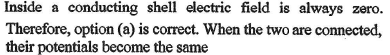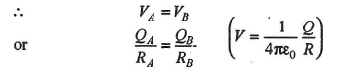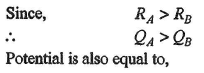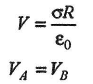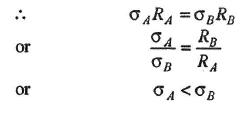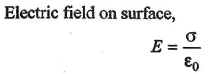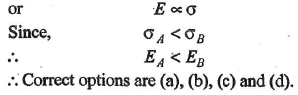QUESTION: 2

### A hollow metal sphere of radius 10 cm is charged such that the potential on its surface becomes 80 V. The potential at the centre of the sphere is

Solution: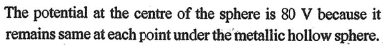QUESTION: 3

### If a charged spherical conductor of radius 10 cm has potential V at a point distant 5 cm from its centre, then the potential at a point distant 15 cm from the centre will be

Solution: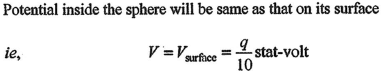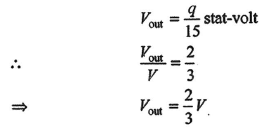QUESTION: 4

Out of two copper  spheres of the same size, x is hollow while y is solid. If they are charged at the same potential, what can be said about the charges on them?

Solution: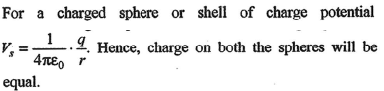QUESTION: 5

The electric potential at centre of metallic conducting sphere is

Solution: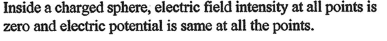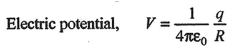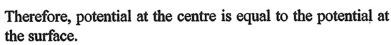QUESTION: 6

Two charged spheres of radii R1 and R2 having equal surface charge density. The ratio of their potential is

Solution:
QUESTION: 7

Consider three concentric shells of metal A,B and C are having radii a,b and c respectively as shown in the figure (a>b>c). Their surface charge densities are σ, -σ and σ respectively. Calculate the electr ic potential on the surface of shell A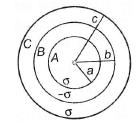Solution: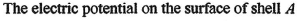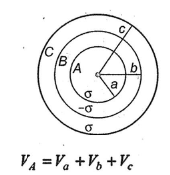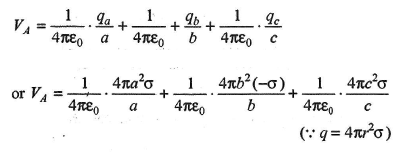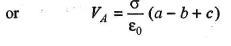QUESTION: 8

A soap bubble is charged to a potential of 16 V. Its radius is, then doubled. The potential of the bubble now will be.

Solution: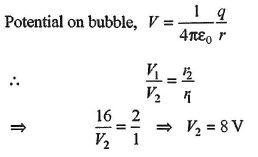QUESTION: 9

Two concentric spheres of radii R and r have similar charges with equal surface densities (σ). What is the electric potential at their common centre?

Solution: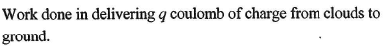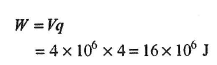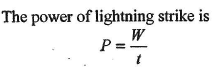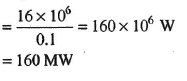QUESTION: 10

Figure shows three spherical and equipotential surfaces A, B and C round a point charge q. The potential difference VA - VB = VB - VC. If t1 and t2  be the distance between them. Then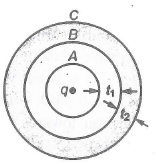Solution: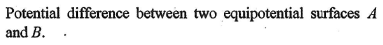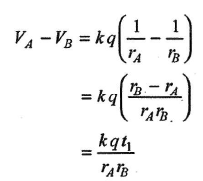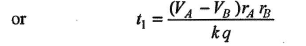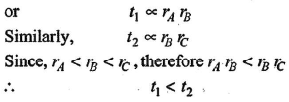QUESTION: 11

A thin spherical conducting shell of radius R has a charge q. Another charge Q is placed at the centre of the shell. The electrostatic potential at a point P at a distance R/2 from the centre of the shell is

Solution: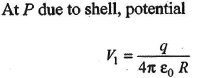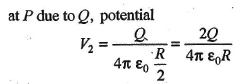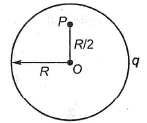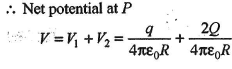QUESTION: 12

The radius of nucleus of silver (alomic number Z = 47 is 3.4 × 10-14 m). The electric potential on the surface of nucleus will be

Solution: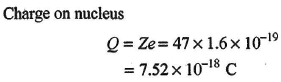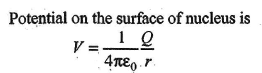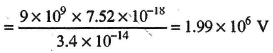*Multiple options can be correct
QUESTION: 13

Which of the following statement (s) is/are correct?

Solution: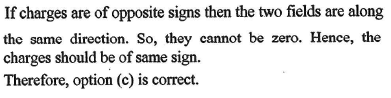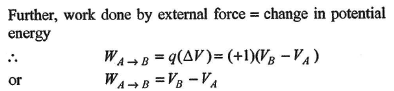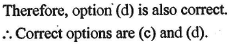QUESTION: 14

The electrostatic potential inside a charged spherical ball is given by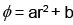where r is the distance from the centre a, b are constants. Then the charge density inside the ball is

Solution: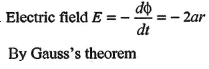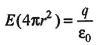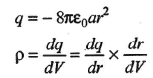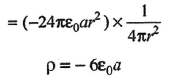QUESTION: 15

Electric charge is uniformly distributed along a long straight wire of radius 1 mm. The charge per cm length of the wire is Q coulomb. Another cylindrical surface of radius 50 cm and length 1 m symmertically encloses the wire. The total electric flux passing through the cylindrical surface is

Solution: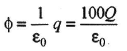QUESTION: 16

The Gaussian surface for calculating the electric field due to a charge distribution is

Solution: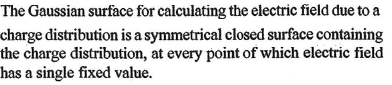QUESTION: 17

A disc of radius a/4 having a uniformly distributed charge 6C is placed in the x-y plane with its centre at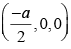A rod of length a carrying a uniformly distributed charge 8 C is placed on the x-axis from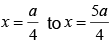. Two point charges -7C and 3C are placed at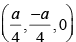and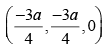respectively. Consider a cubical surface formed by six surfaces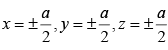. The electric flux through this cubical surface is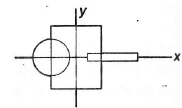Solution: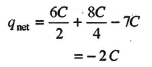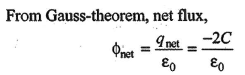QUESTION: 18

The electric charges are distributed in a small volume. The flux of the elctric field through a spherical surface of radius 10 cm surrounding the total charge is 20 Vm.The flux over a concentric sphere of radius 20 cm will be

Solution: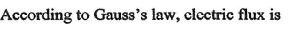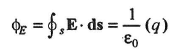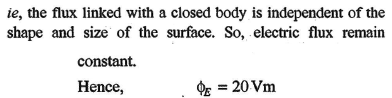QUESTION: 19

Flux coming out from a unit positive charge enclosed in air is

Solution: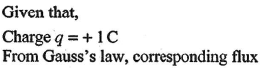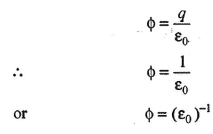QUESTION: 20

What about Gauss theorem is not incorrect?

Solution: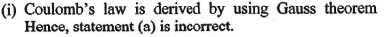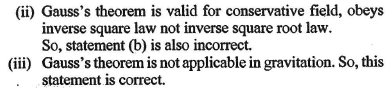QUESTION: 21

A charge q is placed at the corner of a cube of side a. The electric flux through the cube is

Solution: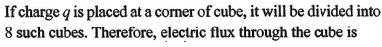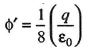QUESTION: 22

Consider the charge configuration and a spherical Gaussian surface as shown in the figure. When calculating the flux of the electric field over the spherical surface, the electric field will be due to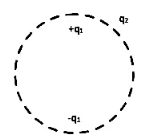Solution: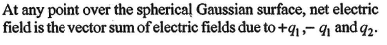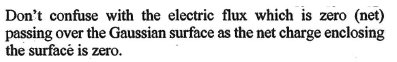QUESTION: 23

A cylinder of radius r and length t is placed in an uniform electric field E parallel to the axis of the cylinder. The total flux for the surface of the cylinder is given by

Solution: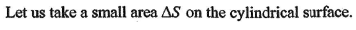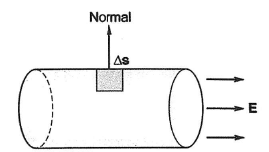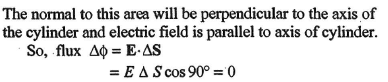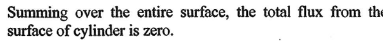QUESTION: 24

The magnitude of electric field at distance r from an infinitely thin rod having a linear charge density λ is (use Gauss's law)

Solution: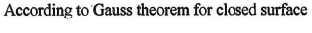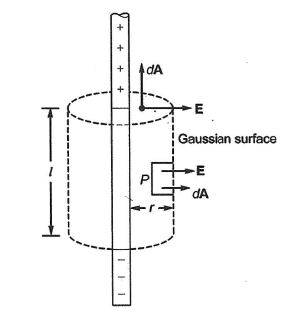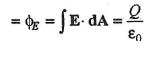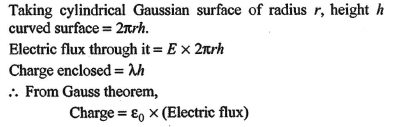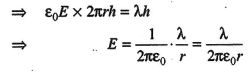QUESTION: 25

Units of electric flux are

Solution: